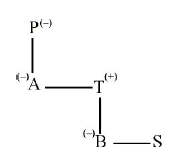## Blood Relation – Practice set 4

D.1-3) Study the following information and answer the given questions.

A and Y are brothers of K. Y is the son of P and S. P is the daughter of X. M is the father-in-law of S. Q is the son of X.

1. How is K related to Q?

a) Cannot be determined

b) Niece

c) Daughter

d) Nephew

e) Son-in-law

b) Niece

B

Explanation

(a) cannot be determined because K has no gender.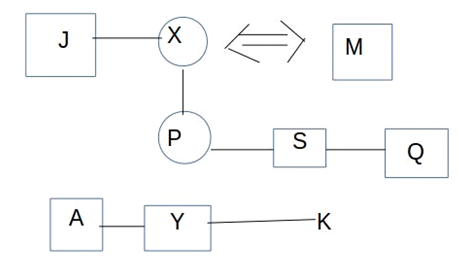2. How is Y related to M?

a) Nephew

b) Father

c) Brother-in-law

d) Grandson

e) Brother

c) Brother-in-law

C

Explanation

(d) Grandson3. If J is brother of X, then how is J related to Q?

a) Uncle

b) Nephew

c) Cannot be determined

d) Brother-in-law

e) Son-in-law

e) Son-in-law

E

Explanation

(a) Uncle4. Kavya says,”kamal’s Father is only son of my father”.How is Kavya related to Kamal?

a) Sister

b) Daughter

c) Cousin

d) Niece

e) Paternal Aunt

e) Paternal Aunt

E

Explanation

e

Only son of my father- deppa brother deepa sister is kamal’s father so deepa is paternal aunt of kamal.

5. Pointing to a man in the photograph,Dhivya said,”his Father’s sister is the only daughter of my Father’s mother”.How is the man’s father related to Dhivya?

a) Father

b) Brother

c) Uncle

d) Grandfather

e) Cousin

c) Uncle

C

Explanation

c

Only daughter of divya’s father mother -Divya’s paternal uncle so the man’s paternal uncle is divya’s paternal uncle thus the man’s father is divya’s uncle

D.6-8) Read the following information carefully to answer the questions given below

‘A+B’ means A is the father of B

‘A – B’ means A is the wife of B

‘A * B’ means A is the brother of B

‘A / B ‘ means A is the daughter of B

6. IF P * R / Q, then which of the following is true?

a) P is the uncle of Q

b) P is the Father of Q

c) P is the brother of Q

d) P is the son of Q

e) None of the above

d) P is the son of Q

D

Explanation

(d)

P * R / Q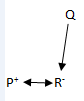7. IF P – R + Q, then which of the following statements is true?

a) P is the mother of Q

b) Q is the daughter of P

c) P is the aunt of Q

d) P is the sister of Q

e) None of the above

a) P is the mother of Q

A

Explanation

(a)

P – R + Q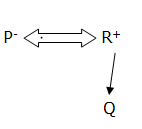8. IF P / R + S + Q, then which of the following is true?

a) P is the daughter of Q

b) Q is the aunt of P

c) P is the aunt of Q

d) P is the mother of Q

e) None of the above

c) P is the aunt of Q

C

Explanation

(c)

P / R+S+Q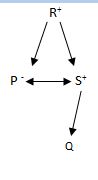D.9-10)

A + B means A is sister of B.

A × B means A is mother of B.

A – B means A is brother of B.

A ÷ B means A is father of B.

9. Which of the following means ‘S’ is paternal aunt of ‘P’ ?

a) S + T + P

b) S + U ÷ P

c) S + U × P

d) S ÷ P + U

e) Other than given options

b) S + U ÷ P

B

Explanation

b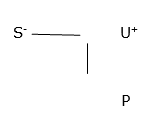10. What will come in place of Question mark (?) If ‘P’ is paternal grandmother of ‘S’ ?

P × A + T ? B + S

a) +

b) ×

c)

d) ÷

e) either ‘×’ or +